Covariance

A measure of the relationship between random variables

What is Covariance?

In mathematics and statistics, covariance is a measure of the relationship between two random variables. The metric evaluates how much – to what extent – the variables change together. In other words, it is essentially a measure of the variance between two variables (note that the variance of one variable equals the variance of the other variable). However, the metric does not assess the dependency between variables.Unlike the correlation coefficient, covariance is measured in units. The units are computed by multiplying the units of the two variables. The variance can take any positive or negative values. The values are interpreted as follows:

• Positive covariance: Indicates that two variables tend to move in the same direction.
• Negative covariance: Reveals that two variables tend to move in inverse directions.

In finance, the concept is primarily used in portfolio theory. One of its most common applications in portfolio theory is the diversification method, using the covariance between assets in a portfolio. By choosing assets that do not exhibit a high positive covariance with each other, the undiversifiable risk can be partially eliminated.

CFI’s Math for Corporate Finance Course explores the financial mathematics concepts required for Financial Modeling.

Formula for Covariance

The covariance formula is similar to the formula for correlation and deals with the calculation of data points from the average value in a dataset. For example, the covariance between two random variables X and Y can be calculated using the following formula (for population):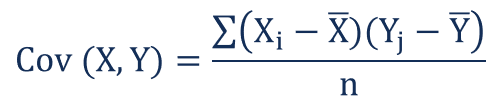For a sample covariance, the formula is slightly adjusted: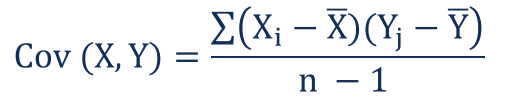Where:

• X– the values of the X-variable
• Yj – the values of the Y-variable
• X̄ – the mean (average) of the X-variable
• Ȳ – the mean (average) of the Y-variable
• n – the number of the data points

Covariance vs. Correlation

Covariance and correlation both primarily assess the relationship between variables. The closest analogy to the relationship between them is the relationship between the variance and standard deviation.

Covariance measures the total variation of two random variables from their expected values. Using covariance, we can only gauge the direction of the relationship (whether the variables tend to move in tandem or show an inverse relationship). However, it does not indicate the strength of the relationship, nor the dependency between the variables.

On the other hand, correlation measures the strength of the relationship between variables. Correlation is the scaled measure of covariance. It is dimensionless. In other words, the correlation coefficient is always a pure value and not measured in any units.

The relationship between the two concepts can be expressed using the formula below: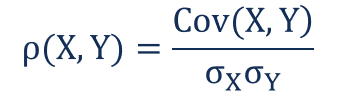Where:

• ρ(X,Y) – the correlation between the variables X and Y
• Cov(X,Y) – the covariance between the variables X and Y
• σ– the standard deviation of the X-variable
• σY – the standard deviation of the Y-variable

Example of Covariance

John is an investor. His portfolio primarily tracks the performance of the S&P 500 and John wants to add the stock of ABC Corp. Before adding the stock to his portfolio, he wants to assess the directional relationship between the stock and the S&P 500.

John does not want to increase the unsystematic risk of his portfolio. Thus, he is not interested in owning securities in the portfolio that tend to move in the same direction.

John can calculate the covariance between the stock of ABC Corp. and S&P 500 by following the steps below:

1. Obtain the data.

First, John obtains the figures for both ABC Corp. stock and the S&P 500. The prices obtained are summarized in the table below: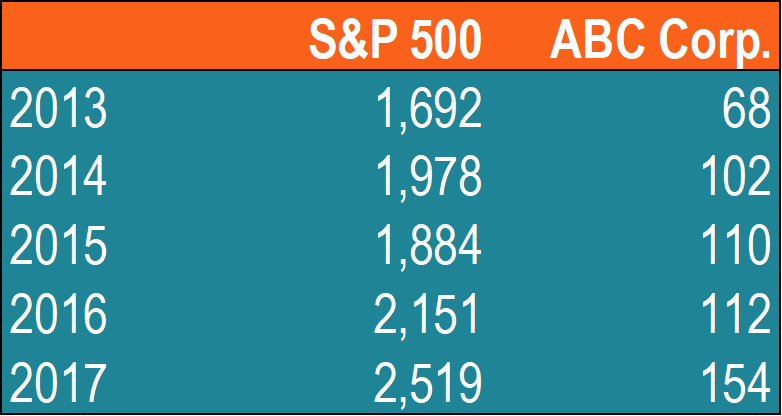2. Calculate the mean (average) prices for each asset.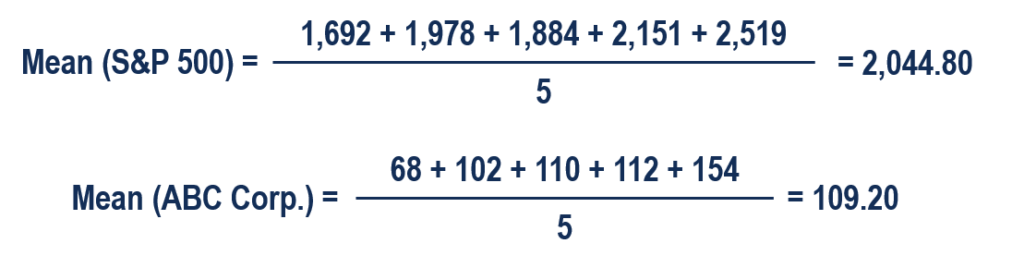3. For each security, find the difference between each value and mean price.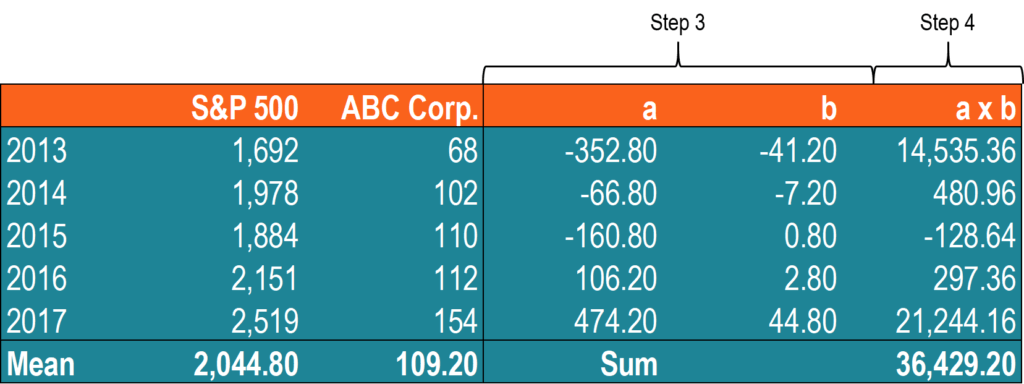4. Multiply the results obtained in the previous step.

5. Using the number calculated in step 4, find the covariance.In such a case, the positive covariance indicates that the price of the stock and the S&P 500 tend to move in the same direction.

CFI offers the Financial Modeling & Valuation Analyst (FMVA)™ certification program for those looking to take their careers to the next level. To keep learning and advancing your career, the following resources will be helpful:

• Investing: A Beginner’s Guide
• Negative Correlation
• Risk and Return
• Risk Management

Financial Analyst Training

Get world-class financial training with CFI’s online certified financial analyst training program!

Gain the confidence you need to move up the ladder in a high powered corporate finance career path.

Learn financial modeling and valuation in Excel the easy way, with step-by-step training.